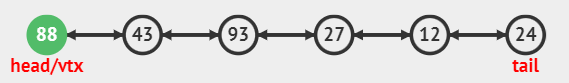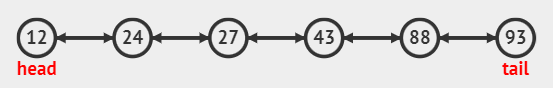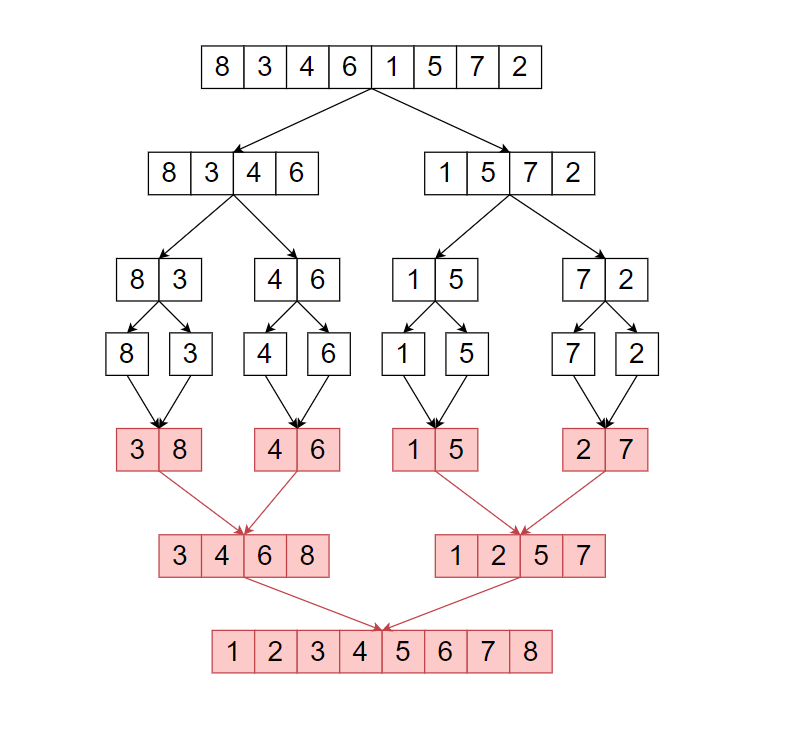# The Merge Sort for Doubly Linked List

## Introduction

Merge Sort and Linked Lists are both hot topics when it comes to tech interviews, But what happens when we mix up these topics and the question that is asked to us is ‘Merge Sort for Doubly Linked List’. Now we know that merge sort is a sorting technique that works on the principle of Divide and Conquer, and a Doubly Linked List is a linked list that contains the address of both its next and previous pointers. Let us now see how we can sort a doubly-linked list using merge sort.

## Problem Statement

The problem that is given to us is fairly simple to understand, we are given an unsorted doubly linked list, and we have to sort it using merge sort.

Let’s say that the linked list that is given to us isThen after applying merge sort to this list, we will get the following as our final answer.## ApproachIn the image above, we can see the functioning of the merge sort. In merge sort, we divide the list into two smaller lists at each step and call the same function recursively on both the smaller parts. From recursion, we get two sorted lists which are then merged by a function, let’s say, merge(), and the final output is a sorted list.

But how will we apply this to doubly linked lists?

The answer is simple. We will first find the middle node of the linked list. You can refer here to find the middle node of a linked list. Then we will split the linked list around the middle node and make two recursive calls on both these sub-linked lists. Finally, we will be merging the two sorted lists (returned from the recursive calls above).

How to code the merge() function?

The function will take two parameters,i.e., ‘FIRST_LIST’ and ‘SECOND_LIST’, and will check for the following cases:

1) If ‘FIRST_LIST’ is NULL, return ‘SECOND_LIST’.

2) If ‘SECOND_LIST’ is null, return ‘FIRST_LIST’.

3) If ‘FIRST_LIST->DATA’ is less than ‘SECOND_LIST->DATA’, then we will append ‘FIRST_LIST’ to the resultant list otherwise we will append ‘SECOND_LIST’.

Now let’s have a look at the final code for better understanding.

## Code

``````#include <iostream>
using namespace std;

// Doubly linked list node class.
class Node
{
public:
int data;
Node *next, *prev;

// Constructor.
Node(int data)
{
this->data = data;
next = NULL;
prev = NULL;
}
};

// This function divides the linked list into two parts and returns the head of second half.
{
while (fastPointer != NULL && fastPointer->next != NULL &&
fastPointer->next->next != NULL)
{
fastPointer = fastPointer->next->next;
slowPointer = slowPointer->next;
}
Node *secondHalf = slowPointer->next;

// Separating the second part.
slowPointer->next = NULL;
return secondHalf;
}

// This function will merge two lists and will return the sorted list.
Node *merge(Node *firstList, Node *secondList)
{
// If the 'firstList' linked list is empty, then we dont have to merge.
if (firstList == NULL)
return secondList;

// If the 'secondList' linked list is empty, then don’t have to merge.
if (secondList == NULL)
return firstList;

// Regular merge conditions.
if (firstList->data > secondList->data)
{
secondList->next = merge(firstList, secondList->next);
secondList->next->prev = secondList;
secondList->prev = NULL;
return secondList;
}
else
{
firstList->next = merge(firstList->next, secondList);
firstList->next->prev = firstList;
firstList->prev = NULL;
return firstList;
}
}

// It returns the sorted doubly linked list.
{
}

}

Node *firstList = NULL, *secondList = NULL;
// Splitting the list.

// Recursively calling merge sort on both the sublists.
secondList = mergeSort(secondList);

// Merging the two sorted sub lists.
Node *sortedList = merge(firstList, secondList);
return sortedList;
}

// Print function.
{
while (temp != NULL)
{
cout << temp->data << " ";
temp = temp->next;
}
}

// Main function.
int main()
{
int n;
cout << "Enter the number of elements in the list: ";
cin >> n;

cout << "Enter the elements: ";
Node *head = NULL, *tail = NULL;

for (int i = 0; i < n; i++)
{
int data;
cin >> data;
Node *newNode = new Node(data);
if (i == 0)
{
}
else
{
tail->next = newNode;
newNode->prev = tail;
tail = newNode;
}
}
cout << "Sorted list: ";

// Calling the 'mergeSort()' function to sort the doubly linked list.

// Printing the final list.
return 0;
}``````

### Input

``````Enter the number of elements in the list: 6
Enter the elements: 8 9 12 2 18 20``````

### Output

``Sorted list: 2 8 9 12 18 20``

### Time Complexity

O(N * log N), where ‘N’ is the length of the array.

Here in the worst case split operation takes O(N), merge operation takes O(N) time and the recursive function will be called O(log N) times so the overall complexity would be (O(N) + O(N)) * O(log(N)) ~ O(N * log(N)).

### Space Complexity

O(log (N)), where ‘N’ is the length of the doubly linked list.

As we are using a recursive function and it would take O(log (N)) space for the recursive stack.

## Key Takeaways

We saw how we could apply merge sort on linked lists and also revised concepts on finding the middle part of and merging doubly-linked lists. Now doubly linked lists is a vast topic, and this was just the beginning of your journey in becoming a star coder. Thus it’s time to move over to our industry-leading practice platform CodeStudio to practice top problemsread interview experiences, and many more. Till then, Happy Coding!